### Home > CC1 > Chapter 1 > Lesson 1.1.3 > Problem1-22

1-22.

For each shape drawn in problem 1-21, choose one of the labels below that best describes that shape. Be as specific as you can. Look in the glossary of this book for more information if you do not remember what one of the words describes.

right triangle

isosceles triangle

square

scalene triangle

rhombus

trapezoid

obtuse triangle

rectangle

hexagon

1.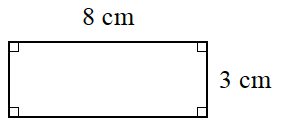This shape has two pairs of
sides of equal lengths.

Notice that all four angles are right angles.

This shape must be a rectangle.

1.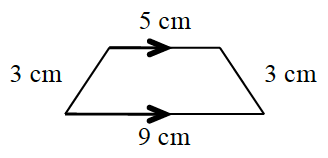Notice that there is one pair
of parallel sides.

This shape must be a trapezoid.

1.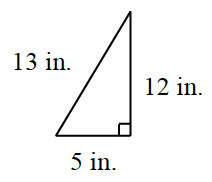This shape has three sides of unequal length.

Notice that there is one
right angle.

This shape must be a right scalene triangle.

1.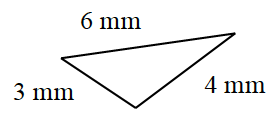All three sides of this triangle are of unequal length.

One of the angles is greater than $90^{\circ}$.

This shape must be an obtuse scalene triangle.

1.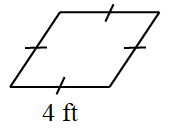Notice that all four sides of this shape are of equal length.

This shape must be a rhombus.

1.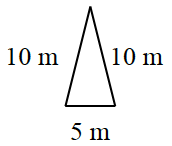Notice that this triangle has
two sides of equal lengths.

This shape must be an isosceles triangle.